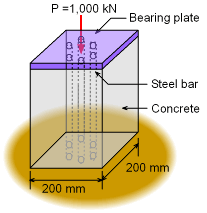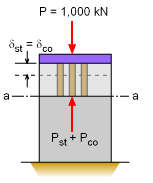Ch 1. Stress and Strain Multimedia Engineering Mechanics NormalStress Shear andBearing Stress NormalStrain Hooke'sLaw ThermalEffects IndeterminateStructures
 Chapter 1. Stress/Strain 2. Torsion 3. Beam Shr/Moment 4. Beam Stresses 5. Beam Deflections 6. Beam-Advanced 7. Stress Analysis 8. Strain Analysis 9. Columns Appendix Basic Math Units Basic Equations Sections Material Properties Structural Shapes Beam Equations Search eBooks Dynamics Fluids Math Mechanics Statics Thermodynamics Author(s): Kurt Gramoll ©Kurt GramollMECHANICS - EXAMPLEReinforced Concrete Post Example A short concrete post is reinforced with six symmetrically placed steel bars so that it can carry a load, P, of 1,000 kN. The concrete post is 200 mm by 200 mm and the cross-sectional area of steel bars is circular. If the allowable stresses of the steel, σst, and concrete, σco, are 120 MPa and 6 MPa, respectively, what is the minimum diameter of the steel bars? Assume the modulus of elasticity of the steel, Est, and and concrete, Eco, are 14 GPa and 200 GPa, respectively. SolutionStatic Equilibrium and Deformation of Steel and Concrete The applied load, P, will be distributed between the steel and the concrete. From equilibrium at an arbitrary section a-a, these forces can be correlated as       ΣF = 0       Pst + Pco = P       Pst + Pco = 1,000 kN       σstAst + σcoAco = 1,000 kN Since no other equations of static equilibrium are available to indicate in what proportion of the load is distributed to each material, the elastic deformation of the structure has to be considered. It is evident from symmetry that the bearing plate causes the steel and concrete to deform equally.      δst = δco       σstLst/Est = σcoLco/Eco Noting that steel and concrete are of equal length and substituting the actual modulus gives      σst/200×106 = σco/14×106      σst = 200 σco/14      σst = 14.29 σco To determine the correct amount of steel required, first assume the concrete will reach it allowable stress limit of 6 MPa. This gives,      σst = 14.29 (6)      σst = 88.74 MPa This indicates that the steel can not be stressed to its limit of 120 MPa without overstressing the concrete. Thus, the assumption that the concrete will fail before the steel is correct. The actual working stresses will be σco = 6 MPa and σco = 88.74 MPa. These values are substituted into the previous static equilibrium equation to obtain      88,740 Ast + 6,000 (0.2)2 = 1,000      88,740 Ast + 240 = 1,000      88,740 Ast = 760      Ast = 8.564×10-3 m2      Ast = 8,564 mm2 The total cross-sectional area, Ast, is the total of the six steel bars. Thus, the area of a single steel bar will be      A = Ast/6      A = 8,564/6 mm2      A = 1,427 mm2      πd2/4 = 1,427 mm2      d2 = 5,708/π mm2      d = 42.63 mm

Practice Homework and Test problems now available in the 'Eng Mechanics' mobile app
Includes over 400 problems with complete detailed solutions.
Available now at the Google Play Store and Apple App Store.